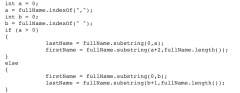• Shuffle
Toggle On
Toggle Off
• Alphabetize
Toggle On
Toggle Off
• Front First
Toggle On
Toggle Off
• Both Sides
Toggle On
Toggle Off
Toggle On
Toggle Off
Front

## Card Range To Study

throughPlay buttonPlay buttonProgress

1/77

Click to flip

Use LEFT and RIGHT arrow keys to navigate between flashcards;

Use UP and DOWN arrow keys to flip the card;

H to show hint;

 The area of a square is stored in a double variable named area. Write an expression whose value is length of one side of the square. Math.sqrt(area) The area of a square is stored in a double variable named area. Write an expression whose value is length of the diagonal of the square. Math.sqrt(area*2) The length of a rectangle is stored in a double variable named length, the width in one named width. Write an expression whose value is the length of the diagonal of the rectangle. Math.sqrt(Math.pow(length,2) + Math.pow(width,2)) If a right triangle has sides of length A, B and C and if C is the largest, then it is called the "hypotenuse" and its length is the square root of the sum of the squares of the lengths of the shorter sides (A and B). Assume that variables a and b have been declared as doubles and that a and b contain the lengths of the shorter sides of a right triangle: write an expression for the length of the hypotenuse. Math.sqrt(Math.pow(a,2) + Math.pow(b,2)) The Math class provides a static method , max, that accepts twoint arguments and returns the value of the larger one. Two int variables ,population1 and population2, have already been declared and initialized . Write an expression (not a statement !) whose value is the larger of population1 and population2 by calling max. Math.max(population1, population2)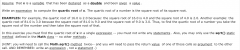Math.sqrt(Math.sqrt(x)) The Math class provides a static method , max, that accepts two int arguments and returns the value of the larger one. Four int variables , population1, population2, population3, and population4 have already been declared and initialized . Write an expression (not a statement !) whose value is the largest of population1, population2, population3, and population4 by calling max. Math.max(Math.max(population1,population2),Math.max(population3,population4)) Write a character literal representing the (upper case) letter A. 'A' Write a character literal representing a comma. ',' Write a character literal representing the digit 1. '1' Write a literal representing the character whose unicode value is 65. 'A' Write a literal representing the largest character value . 65535 The character escape sequence to force the cursor to go to the next line is: \n The character escape sequence to force the cursor to advance forward to the next tab setting is: \t The character escape sequence to represent a single quote is \' The character escape sequence to represent a double quote is: \" Declare a character variable named c. char c; Java represents characters using Unicode Write a String constant consisting of exactly 5 exclamation marks. "!!!!!" Write a String constant consisting of exactly one character -- any character will do. "d" Write a String constant that is the empty string . "" Declare a String variable named mailingAddress. String mailingAddress = new String(); Write the declaration of a String variable named title. String title = new String(); Write the declaration of three String variables named win, place, and show. String win = new String(); String place = new String(); String show = new String(); Write the declaration of a String variable named foreground and initialize it to "black". String foreground = new String("black"); Declare a String variable named oneSpace, and initialize it to a String consisting of a single space. String oneSpace = " "; Write the declaration of two String variable named background and selectionColor and initialize them to "white" and "blue" respectively. String background="white"; String selectionColor="blue"; Declare a String variable named empty, and initialize it to the empty String . String empty = new String(); Assume that word is a String variable . Write a statement to display the message "Today's Word-Of-The-Day is: " followed by the value of word. The message and the value of word should appear together, on a single line on standard output . System.out.println("Today's Word-Of-The-Day is: " + word); Assume that message is a String variable . Write a statement to display its value on standard output . System.out.println(message); Assume that the String variable named foreground has already been declared . Assign it the value "red". foreground = "red"; Assume that theString variable named text has already been declared . Assign to it the empty string . text = ""; There are two String variables , s1 and s2, that have already been declared and initialized . Write some code that exchanges their values . Declare any other variables as necessary. String temp = new String(); temp = s1; s1 = s2; s2 = temp; Given three String variables that have been declared and given values , firstName, middleName, and lastName, write an expression whose value is the values of each these variables joined by a single space. So if firstName, middleName, and lastName, had the values "Big", "Bill", and "Broonzy", the expression 's value would be "Big Bill Broonzy". Alternatively, if firstName, middleName, and lastName, had the values "Jerry", "Lee", and "Lewis", the expression 's value would be "Jerry Lee Lewis firstName + " " + middleName + " " + lastName Given a String variable address, write a String expression consisting of the string "http://" concatenated with the variable 's String value . So, if the variable refers to "www.turingscraft.com", the value of the expression would be "http://www.turingscraft.com". "http://" + address Write an expression that concatenates the String variable suffix onto the end of the String variable prefix . prefix + suffix Given a String variable word, write a String expression that parenthesizes the value of word. So, if word contains "sadly", the value of the expression would be the String "(sadly)" "(" + word + ")" Given four int variables that have been declared and given values , octet1, octet2, octet3, and octet4, write a String expression whose value is the String equivalent of each these variables joined by a single period (.) So if octet1, octet2, octet3, and octet4, had the values 129, 42, 26, and 212 the expression 's value would be "129.42.26.212". Alternatively, if octet1, octet2, octet3, and octet4, had the values 192, 168, 1and 44 the expression 's value would be "192.168.1.44". octet1 + "." + octet2 + "." + octet3 + "." + octet4 Given three String variables that have been declared and given values , gold, silver, and bronze, write an expression whose value is the values of each these variables joined by a newline character . So if gold, silver, and bronze, had the values "Arakawa", "Cohen", and "Slutskaya", the expression , if it were printed would have the names "Arakawa", "Cohen", and "Slutskaya" each appearing on a separate line. (Do NOT print anything in this exercise: just write the expression .) gold + "\n" + silver + "\n" + bronze Given three int variables that have been declared and given values , areaCode, exchange, and lastFour, write a String expression whose value is the String equivalent of each these variables joined by a single hyphen (-) So if areaCode, exchange, and lastFour, had the values 800, 555, and 1212, the expression 's value would be "800-555-1212". Alternatively, if areaCode, exchange, and lastFour, had the values 212, 867 and 5309 the expression 's value would be "212-867-5309". areaCode + "-" + exchange + "-" + lastFour Write a statement that reads a word from standard input into firstWord. Assume that firstWord. has already been declared as a String variable . Assume also that stdin is a variable that references a Scanner object associated with standard input. firstWord = stdin.next(); Assume that name and age have been declared suitably for storing names (like "Abdullah", "Alexandra" and "Zoe") and ages respectively. Assume also that stdin is a variable that references a Scanner object associated with standard input. Write some code that reads in a name and an age and then prints the message "The age of NAME is AGE" on a line by itself, where NAME and AGE are replaced by the values read in for the variables name and age. For example, if your code read in "Rohit" and 70 then it would print out "The age of Rohit is 70" on a line by itself. There should NOT be a period in the output . name = stdin.next(); age = stdin.nextInt(); System.out.println("The age of " + name + " is " + age); Three business partners are forming a company whose name will be of the form "Name1, Name2 and Name3". However, they can't agree whose name should be first, second or last. Help them out by writing code that reads in their three names and prints each possible combination exactly once, on a line by itself (that is, each possible combination is terminated with a newline character ). Assume that name1, name2 and name3 have already been declared and use them in your code. Assume also that stdin is a variable that references a Scanner object associated with standard input. For example, if your code read in "Larry", "Curly" and "Moe" it would print out "Larry, Curly and Moe", "Curly, Larry and Moe", etc., each on a separate line. name1 = stdin.next(); name2 = stdin.next(); name3 = stdin.next(); System.out.println(name1 + ", " + name2 + " and " + name3); System.out.println(name1 + ", " + name3 + " and " + name2); System.out.println(name2 + ", " + name1 + " and " + name3); System.out.println(name2 + ", " + name3 + " and " + name1); System.out.println(name3 + ", " + name2 + " and " + name1); System.out.println(name3 + ", " + name1 + " and " + name2); Assume that name has been declared suitably for storing names (like "Amy", "Fritz" and "Moustafa"). Assume also that stdin is a variable that references a Scanner object associated with standard input. Write some code that reads a value into name then prints the message "Greetings, NAMEVALUE!!!" on a line by itself where NAMEVALUE is replaced the value that was read into name . For example, if your code read in "Hassan" it would print out "Greetings, Hassan!!!" on a line by itself. name = stdin.next();System.out.println("Greetings, " + name + "!!!"); Assume that name has been declared suitably for storing names (like "Misha", "Emily" and "Sofia"). Assume also that stdin is a variable that references a Scanner object associated with standard input Write some code that reads a value into name then prints the message "Greetings, NAME " on a line by itself, where NAME is replaced the value that was read into name . For example, if your code read in "Rachel" it would print out "Greetings, Rachel" on a line by itself. name = stdin.next();System.out.println("Greetings, " + name); Given a String variable named sentence that has been initialized , write an expression whose value is the number of characters in the String referred to by sentence. sentence.length() Assume that name is a variable of type String that has been assigned a value . Write an expression whose value is the first character of the value of name . So if the value of name were "Smith" the expression 's value would be 'S'. name.charAt(0) Given the String variable str, write an expression that evaluates to the character at index 0 of str. str.charAt(0) Given the String variable name , write an expression that evaluates to the third character of name . name.charAt(2) Assume that name is a variable of type String that has been assigned a value . Write an expression whose value is the second character of the value of name . So if the value of name were "Smith" the expression 's value would be 'm'. name.charAt(1) Write an expression that whose value is the fifth character of the String name . name.charAt(4) Assume that name is a variable of type String that has been assigned a value . Write an expression whose value is the last character of the value of name . So if the value of name were "Blair" the expression 's value would be 'r'. name.charAt(name.length() - 1) Write an expression that evaluates to true if and only if the string variable s equals the string "end". s.equals("end") Write an expression that evaluates to true if the value of the string variable s1 is greater than the value of string variable s2. s1.compareTo(s2)>0 Write an expression that evaluates to true if the value of variable lastName is greater than the string Dexter. lastName.compareTo("Dexter")>0 Write an expression that evaluates to true if and only if the string variable s does not equal the String literal end. (!s.equals("end")) Given the String variables name1 and name2, write a fragment of code that assigns the larger of the two to the variable first (assume that all three are already declared and that name1 and name2 have been assigned values ). (NOTE: "larger " here means alphabetically larger , not "longer". Thus, "mouse" is larger than "elephant" because "mouse" comes later in the dictionary than "elephant"!)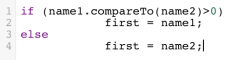Given the string variables name1, name2, and name3, write a fragment of code that assigns the largest value to the variable max (assume all three have already been declared and have been assigned values ).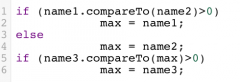Assume thats is a String . Write an expression whose value is true if and only if the value ofs would come between "mortgage" and "mortuary" in the dictionary. (s.compareTo("mortgage")>0 && s.compareTo("mortuary")<0) Clunker Motors Inc. is recalling all vehicles in its Extravagant line from model years 1999-2002. Given a variable modelYear and a String modelName write a statement that prints the message "RECALL" to standard output if the values ofmodelYear andmodelName match the recall details.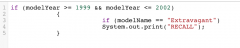Clunker Motors Inc. is recalling all vehicles in its Extravagant line from model years 1999-2002 as well all vehicles in its Guzzler line from model years 2004-2007. Given a variable modelYear and a String modelName write a statement that prints the message "RECALL" to standard output if the values ofmodelYear andmodelName match the recall details.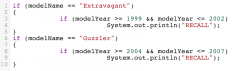Clunker Motors Inc. is recalling all vehicles in its Extravagant line from model years 1999-2002. A boolean variable named recalled has been declared . Given a variable modelYear and a String modelName write a statement that assigns true to recalled if the values ofmodelYear andmodelName match the recall details and assigns false otherwise.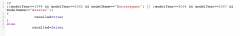Clunker Motors Inc. is recalling all vehicles in its Extravagant line from model years 1999-2002 as well all vehicles in its Guzzler line from model years 2004-2007. A boolean variable named recalled has been declared . Given a variable modelYear and a String modelName write a statement that assigns true torecalled if the values ofmodelYear andmodelName match the recall details and assigns false otherwise.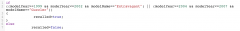Given the String variable address, write an expression that returns the position of the first occurrence of the String "Avenue" in address. address.indexOf("Avenue") Assume that name is a variable of type String that has been assigned a value . Write an expression whose value is a String containing the first character of the value of name . So if the value of name were "Smith" the expression 's value would be "S". name.substring(0,1) Assume that name is a variable of type String that has been assigned a value . Write an expression whose value is a String containing the second character of the value of name . So if the value of name were "Smith" the expression 's value would be "m". name.substring(1,2) Write an expression that results in a String consisting of the third through tenth characters of the String s. s.substring(2,10) Assume that word is a variable of type String that has been assigned a value . Write an expression whose value is a String consisting of the last three characters of the value of word. So if the value of word were "biggest" the expression 's value would be "est". word.substring(word.length() - 3,word.length()) Given three String variables that have been declared and given values , firstName, middleName, and lastName, write an expression whose value is the initials of the three names : the first letter of each, joined together. So if firstName, middleName, and lastName, had the values "John", "Fitzgerald", and "Kennedy", the expression 's value would be JFK". Alternatively, if firstName, middleName, and lastName, had the values "Franklin", "Delano", and "Roosevelt", the expression 's value would be "FDR". "" + firstName.charAt(0) + middleName.charAt(0) + lastName.charAt(0) Assume that sentence is a variable of type String that has been assigned a value . Assume furthermore that this value is a String consisting of words separated by single space characters with a period at the end. For example: "This is a possible value of sentence." Assume that there is another variable declared , firstWord, also of type String . Write the statements needed so that the first word of the value of sentence is assigned to firstWord. So, if the value of sentence were "Broccoli is delicious." your code would assign the value "Broccoli" to firstWord. firstWord = sentence.substring(0,sentence.indexOf(' ')); Assume that given , middle and family are three variables of type String that have been assigned values . Write an expression whose value is a String consisting of the first character of given followed by a period followed by the first character of middle followed by a period followed by the first character of family followed by a period: in other words, the initials of the name . So if the values of these three variables were "John" "Fitzgerald" "Kennedy", then the expression 's value would be "J.F.K.". given.charAt(0) + "." + middle.charAt(0) + "." + family.charAt(0) + "." Given a String variable named sentence that has been initialized , write an expression whose value is the the very last character in the String referred to by sentence. sentence.charAt(sentence.length() - 1) Write a sequence of statements that finds the first comma in the String line, and assigns to the variable clause the portion of line up to, but not including the comma. You may assume that an int variable pos, as well as the variables line and clause, have already been declared . pos = line.indexOf(','); clause = line.substring(0,pos); Assume that sentence is a variable of type String that has been assigned a value . Assume furthermore that this value is a String consisting of words separated by single space characters with a period at the end. For example: "This is a possible value of sentence." Assume that there is another variable declared , secondWord, also of type String . Write the statements needed so that the second word of the value of sentence is assigned to secondWord. So, if the value of sentence were "Broccoli is delicious." your code would assign the value "is" to secondWord. int start = sentence.indexOf(" ") + 1; int end = sentence.indexOf(" ", start+1); secondWord = sentence.substring(start,end); Given a String variable named sentence that has been initialized , write an expression whose value is the index of the very last character in the String referred to by sentence. sentence.length() - 1 Assume that name is a variable of type String that has been assigned a value . Write an expression whose value is a String containing the last character of the value of name . So if the value of name were "Smith" the expression 's value would be "h". name.substring(name.length() - 1,name.length()) A String variable , fullName, contains a name in one of two formats: last name , first name (comma followed by a blank), orfirst name last name (single blank) Extract the first name into the String variable firstName and the last name into the String variable lastName. Assume the variables have been declared and fullName already initialized . You may also declare any other necessary variables .